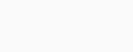# Check a number is Narcissistic number or not in Python

Hello Coders, this tutorial deals with a program to check whether a number is a Narcissistic number or not in Python.
Let’s start. We will see a simple example here to understand clearly.

## Check Narcissistic number in Python

Narcissistic numbers are the special type of numbers where that number can be formed by sum of its own digits raised to the power of no. of digits.

example:
153= 1**(no. of digits in that number) + 5**(no. of digits in that number) + 3**(no. of digits in that number) = 1**3+5**3+**3=153

These types of numbers are termed as Narcissistic numbers.

Algorithm to check Narcissistic number or not

1. Taking input in a variable named ‘n’ and typecasting and storing in another variable ‘m’.
2. Under while loop calculating the sum of individual digits raised to the power of no. of digits in the number.
3. Finally checking whether the calculated number is equal to the given input number or not if ‘Yes’ then it is a Narcissistic number else ‘No’ it is not a Narcissistic number.

Let us see the code:

```n=input()
m=int(n)
s=0
q=m
while(m!=0):
p=m%10
s+=p**(len(n))
m=m//10
if(s==q):
print('Yes')
else:
print('No')```

Output:For any queries please comment below.